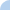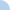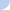# Newton's Laws and Momentum Exam

Weight=Mass x Gravity w = mg
Force=Mass x Accelertion F = ma
Momentum=mass x Velocity p = mvFirst name Last nameThis activity was created by a Quia Web subscriber. Learn more about QuiaCreate your own activities